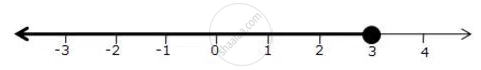Share

Represent the Following Inequalities on Real Number Lines 2(2x- 3) <= 6 - ICSE Class 10 - Mathematics

ConceptLinear Inequations in One Unknown

Question

Represent the following inequalities on real number lines

2(2x- 3) <= 6

Solution

2(2x - 3) <= 6

2x - 3 <= 3

2x <= 6

x <= 3

Solution on number line isIs there an error in this question or solution?

APPEARS IN

Solution Represent the Following Inequalities on Real Number Lines 2(2x- 3) <= 6 Concept: Linear Inequations in One Unknown.
S